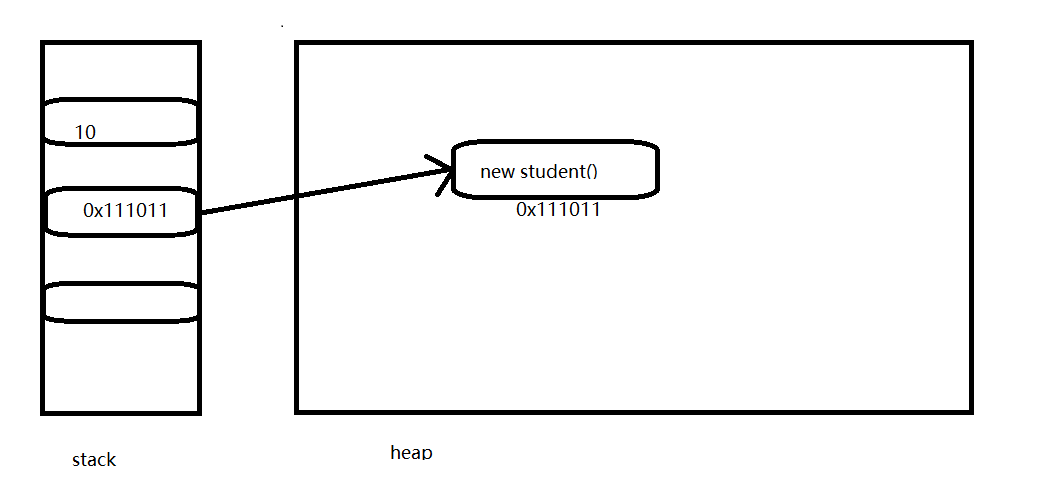### c#入参使用引用类型为啥要加ref?### 引用类型添加ref的作用是啥？

``````Student student1 = new Student("Jack");
Student student2 = new Student("Lucy");
WithoutRef(student1);
WithRef(ref student2);
Console.WriteLine(\$"不带ref的方法---{student1.Name}");
Console.WriteLine(\$"带ref的方法---{student2.Name}");

//不带ref的方法
static void WithoutRef(Student stu)
{
stu.Name = "Bruce";
}

//不带ref的方法
static void WithRef(ref Student stu)
{
stu.Name = "Bruce";
}

public class Student
{
public Student(string name)
{
Name = name;

}
public string Name { get; set; }
}
````````````Student student1 = new Student("Jack");
Student student2 = new Student("Lucy");
WithoutRef(student1);
WithRef(ref student2);
Console.WriteLine(\$"不带ref的方法---{student1.Name}");
Console.WriteLine(\$"带ref的方法---{student2.Name}");

//不带ref的方法
static void WithoutRef(Student stu)
{
stu = new Student("Bruce");
}

//不带ref的方法
static void WithRef(ref Student stu)
{
stu = new Student("Bruce");
}

public class Student
{
public Student(string name)
{
Name = name;

}
public string Name { get; set; }
}
``````ref传递的是栈地址

Tags: